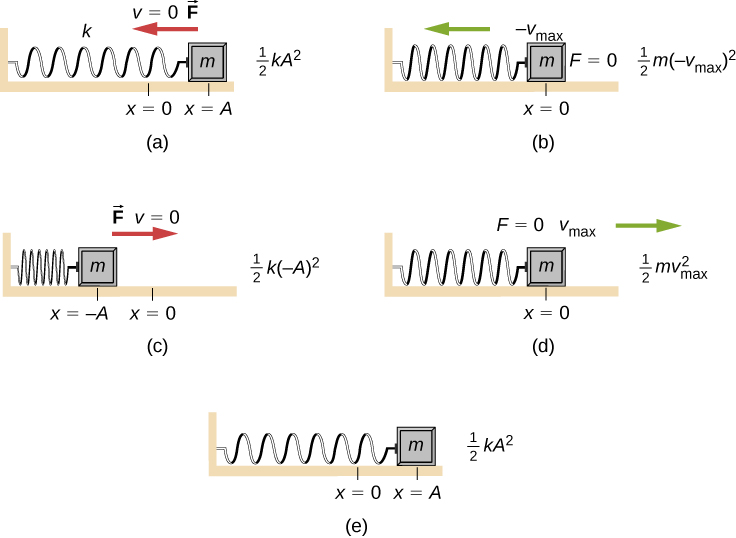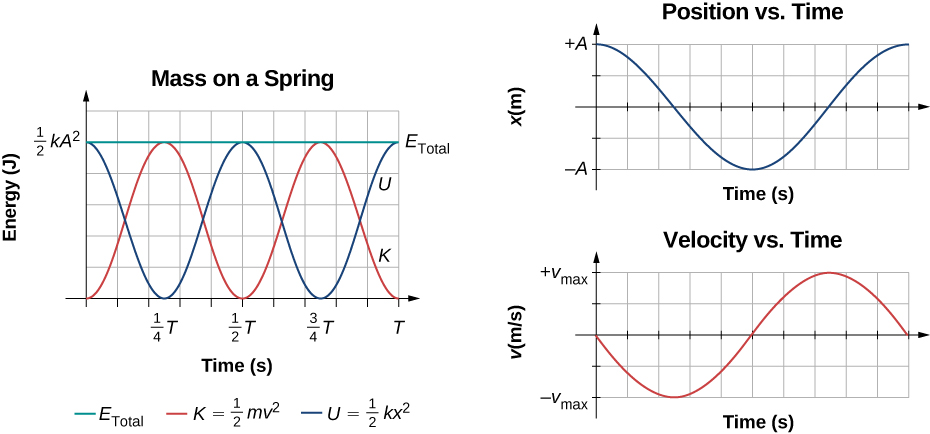# 15.2 Energy in simple harmonic motion  (Page 2/8)

 Page 2 / 8The transformation of energy in SHM for an object attached to a spring on a frictionless surface. (a) When the mass is at the position x = + A , all the energy is stored as potential energy in the spring U = 1 2 k A 2 . The kinetic energy is equal to zero because the velocity of the mass is zero. (b) As the mass moves toward x = − A , the mass crosses the position x = 0 . At this point, the spring is neither extended nor compressed, so the potential energy stored in the spring is zero. At x = 0 , the total energy is all kinetic energy where K = 1 2 m ( − v max ) 2 . (c) The mass continues to move until it reaches x = − A where the mass stops and starts moving toward x = + A . At the position x = − A , the total energy is stored as potential energy in the compressed U = 1 2 k ( − A ) 2 and the kinetic energy is zero. (d) As the mass passes through the position x = 0 , the kinetic energy is K = 1 2 m v max 2 and the potential energy stored in the spring is zero. (e) The mass returns to the position x = + A , where K = 0 and U = 1 2 k A 2 .

Consider [link] , which shows the energy at specific points on the periodic motion. While staying constant, the energy oscillates between the kinetic energy of the block and the potential energy stored in the spring:

${E}_{\text{Total}}=U+K=\frac{1}{2}k{x}^{2}+\frac{1}{2}m{v}^{2}.$

The motion of the block on a spring in SHM is defined by the position $x\left(t\right)=A\text{cos}\left(\omega t+\varphi \right)$ with a velocity of $v\left(t\right)=\text{−}A\omega \text{sin}\left(\omega t+\varphi \right)$ . Using these equations, the trigonometric identity ${\text{cos}}^{2}\theta +{\text{sin}}^{2}\theta =1$ and $\omega =\sqrt{\frac{k}{m}}$ , we can find the total energy of the system:

$\begin{array}{cc}\hfill {E}_{\text{Total}}& =\frac{1}{2}k{A}^{2}{\text{cos}}^{2}\left(\omega t+\varphi \right)+\frac{1}{2}m{A}^{2}{\omega }^{2}{\text{sin}}^{2}\left(\omega t+\varphi \right)\hfill \\ & =\frac{1}{2}k{A}^{2}{\text{cos}}^{2}\left(\omega t+\varphi \right)+\frac{1}{2}m{A}^{2}\left(\frac{k}{m}\right){\text{sin}}^{2}\left(\omega t+\varphi \right)\hfill \\ & =\frac{1}{2}k{A}^{2}{\text{cos}}^{2}\left(\omega t+\varphi \right)+\frac{1}{2}k{A}^{2}{\text{sin}}^{2}\left(\omega t+\varphi \right)\hfill \\ & =\frac{1}{2}k{A}^{2}\left({\text{cos}}^{2}\left(\omega t+\varphi \right)+{\text{sin}}^{2}\left(\omega t+\varphi \right)\right)\hfill \\ & =\frac{1}{2}k{A}^{2}.\hfill \end{array}$

The total energy of the system of a block and a spring is equal to the sum of the potential energy stored in the spring plus the kinetic energy of the block and is proportional to the square of the amplitude ${E}_{\text{Total}}=\left(1\text{/}2\right)k{A}^{2}.$ The total energy of the system is constant.

A closer look at the energy of the system shows that the kinetic energy oscillates like a sine-squared function, while the potential energy oscillates like a cosine-squared function. However, the total energy for the system is constant and is proportional to the amplitude squared. [link] shows a plot of the potential, kinetic, and total energies of the block and spring system as a function of time. Also plotted are the position and velocity as a function of time. Before time $t=0.0\phantom{\rule{0.2em}{0ex}}\text{s,}$ the block is attached to the spring and placed at the equilibrium position. Work is done on the block by applying an external force, pulling it out to a position of $x=+A$ . The system now has potential energy stored in the spring. At time $t=0.00\phantom{\rule{0.2em}{0ex}}\text{s,}$ the position of the block is equal to the amplitude, the potential energy stored in the spring is equal to $U=\frac{1}{2}k{A}^{2}$ , and the force on the block is maximum and points in the negative x -direction $\left({F}_{S}=\text{−}kA\right)$ . The velocity and kinetic energy of the block are zero at time $t=0.00\phantom{\rule{0.2em}{0ex}}\text{s}\text{.}$ At time $t=0.00\phantom{\rule{0.2em}{0ex}}\text{s,}$ the block is released from rest.Graph of the kinetic energy, potential energy, and total energy of a block oscillating on a spring in SHM. Also shown are the graphs of position versus time and velocity versus time. The total energy remains constant, but the energy oscillates between kinetic energy and potential energy. When the kinetic energy is maximum, the potential energy is zero. This occurs when the velocity is maximum and the mass is at the equilibrium position. The potential energy is maximum when the speed is zero. The total energy is the sum of the kinetic energy plus the potential energy and it is constant.

find the angle of projection at which the horizontal range is twice the maximum height of a projectile
impulse by height fomula
Hello
what is impulse?
impulse is the integral of a force (F),over the interval for which it act
Agbeyangi
What is significance of vector?
magnitude and direction
Chris
what is impulse?
the product of force and time
Robert
find the flow rate of a fluid of viscosity 0.0015N-s/m³ flowing through a pipe of radius 50cm and length 100m at a pressure differential of 200000N/m²
friend ,what is the equation your question
lasitha
What is victor
How are you all?
what is the formula for momentum?
p=mv
Grant
what is the formula for angular displacement ?
lasitha
angular displacement = l/r
anand
friend ,what is the " l "?
lasitha
I have another problem,what is the difference between pure chemistry and applied chemistry ?
lasitha
l stands for linear displacement and l>>r
anand
r stands for radius or position vector of particle
anand
pure chemistry is related to classical and basic concepts of chemistry while applied Chemistry deals with it's application oriented concepts and procedures.
anand
what is the deference between precision and accuracy?
ShAmy
I think in general both are same , more accuracy means more precise
anand
and lessor error
anand
friends,how to find correct applied chemistry notes?
lasitha
what are the main concepts of applied chemistry ?
lasitha
anand,can you give an example for angular displacement ?
lasitha
when any particle rotates or revolves about something , an axis or point ,then angle traversed is angular displacement
anand
like a revolving fan
anand
yeah ,I understood now, thanks!
lasitha
o need this application in French language
Why is it that the definition is not there
Bello
what is physics
the study of matter in relation to energy
Robert
Ampher law
Zia
how do convert acceleration
multiply by 3.6
Robert
a man drops a rock into a wall
then
Robert
how did John Dalton contribute to A.theory?
Angela
Atomic theory,
Angela
according to John Dalton all the matter was composed of atom and indivisible, indestructible building blocks. while the atom of different elements have different mass and size.
Rakesh
two parallel wires are 5.80cm apart and carry currents in opposite direction , as shown in figure .find the magnitude and direction of the magnetic field at point p due to two 1.50mm segments of wire that are opposite each other and each 8.00 cm from p.
solve it
Barilee
how
Alia
give me its solution
Alia
where is divergence law
.
jagan
the formula velocity tell me
Zakariye
v=d/t
Robert
in electrostatics
HarishByByByBy